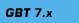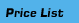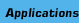Geometric Bounding Toolbox (GBT 7.3) for MATLAB GBT is snow available in stand-alone program which directly runs on Windows 95, 98, 2000, NT, XP without any MATLAB installation. Compared to visual design tools the advantage of GBT is that it allows definitions of objects by numerical/algebraic formulae which reduces effort. Also objects sizes will be precise and description data can be easily stored. Non-convex and disconnected objects can now be handled by a single name.Here are some illustrations which were computed with less than 3 lines of MATLAB code:Version 7 of GBT is a complete rewrite of GBT 6. The latest version GBT 7.3 can now compute with sets of nonconvex polyhedral complexes or polygons. The latest improvements are summarized in "New Features of GBT 7.3". The novelties of GBT 7 from GBT 6 are summarized here. Geometric Bounding Toolbox (GBT) is now used by many scientists, teachers, engineers. It provides a simple to use software in the powerful MATLAB environment to perform operations on complex-shape geometric objects. GBT is unique since: There is no other package that would provide you with the accurate numerical details of your geometric computations on complex non-convex bodies with the same ease ! There is no other comprehensive package that can handle more than 3D with all the operations of algebraic sum/difference set union/difference, volume and surface area computation for complex non-convex shapes obtained with single instructions. And you can operate on objects in 4D, 5D, 6D,... ! GBT is snow available in stand-alone code which directly runs on Windows 95, 98, 2000, NT, XP without any MATLAB installation . As an ilustration consider a dodecahedron and a half-space given in 3D, known only by their names DODE and HF1. A simple instruction can produce the cut of the dodecahedron with the half-space as follows: Given a dodecahedron named DODE...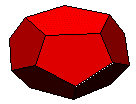to obtain its cut by a given half-space HF1 can simply be done by the command DODE1=INTERSCT(DODE,HF1)...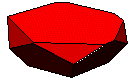...and cut by another half-space HF2 is simply by DODE2=INTERSCT(DODE1, HF2)...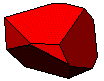There is no need to remember the details of vertices, edges and facets. For instance the volume of the final object can be obtained simply by typing V1=POLVOL(DODE2) ... or its diameter by D1=DIAMETR(DODE2) or its extremum point in a given direction DIR1 by VERTEX1=DIREXT(DIR1,DODE2) which is rather handy in optimisation problems.(See List of routines of GBT 7.2.) The features of objects is handled by the package internally. You can easily extract only those numerical details that you are really interested in (vertices, edges, facets, etc.) Operations can be performed with the same ease on ellipsoids (including the 2D ellipses). Version 7.3 of GBT can compute the volume or area of sets of nonconvex polyhedral complexes and operations of union, intersection and others con be performed. The upgrade is summarized here.